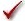## Please enable JavaScript to view this site.

 Navigation: PRINCIPLES OF STATISTICS > Analysis checklists Analysis checklist: Repeated measures two-way ANOVA (and mixed model)

Two-way ANOVA, also called two-factor ANOVA, determines how a response is affected by two factors. "Repeated measures" means that one of the factors was repeated. For example you might compare two treatments, and measure each subject at four time points (repeated). Read elsewhere to learn about choosing a test, graphing the data, and interpreting the results.

###Are the data matched?

If the matching is effective in controlling for experimental variability, repeated-measures ANOVA will be more powerful than regular ANOVA. Also check that your choice in the experimental design tab matches how the data are actually arranged. If you make a mistake, and the calculations are done assuming the wrong factor is repeated, the results won't be correct or useful.

###Are there two factors?

One-way ANOVA compares three or more groups defined by one factor. For example, you might compare a control group with a drug treatment group and a group treated with drug plus antagonist. Or you might compare a control group with five different drug treatments. Prism has a separate analysis for one-way ANOVA.

Some experiments involve more than two factors. For example, you might compare three different drugs in men and women at four time points. There are three factors in that experiment: drug treatment, gender and time. These data need to be analyzed by three-way ANOVA, also called three-factor ANOVA.

###Are both factors “fixed” rather than “random”?

While Prism assumes the participants in repeated measures are chosen randomly, it assumes  that the treatments or categories designated by rows or data set columns are fixed. This means you are asking about how those particular treatments or categories affect the results. Different calculations would be needed if you randomly selected the treatments or categories from an infinite (or at least large) number of possible treatments or categories, and want to reach conclusions about differences among ALL the treatments or categories, even the ones you didn't include in this experiment. Prism does not handle this situation.

###Can you accept the assumption of sphericity?

A random factor that causes a measurement in one subject to be a bit high (or low) should have no effect on the next measurement in the same subject. This assumption is called circularity or sphericity. It is closely related to another term you may encounter in advanced texts, compound symmetry.

You only have to worry about the assumption of circularity when your experiment truly is a repeated-measures experiment, with measurements from a single subject. Circularity is unlikely to be an issue with randomized block experiments where you used a matched set of subjects (or a matched set of experiments)

Repeated-measures ANOVA is quite sensitive to violations of the assumption of circularity. If the assumption is violated, the P value will be too low. You'll violate this assumption when the repeated measurements are made too close together so that random factors that cause a particular value to be high (or low) don't wash away or dissipate before the next measurement. To avoid violating the assumption, wait long enough between treatments so the subject is essentially the same as before the treatment. Also randomize the order of treatments, when possible.